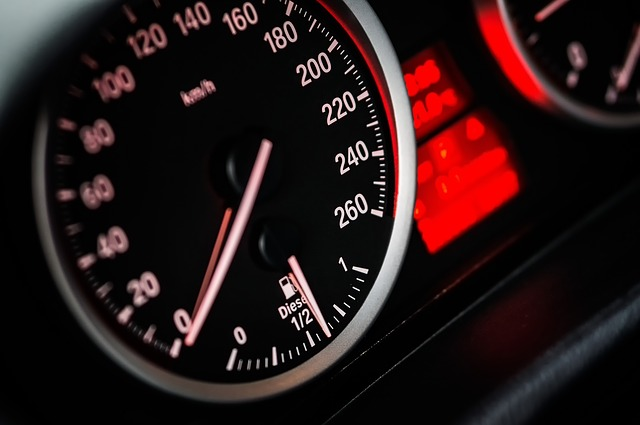# What are Speed, Distance Time Questions?

Typically, these questions involve something moving at a constant speed. From the three variables (speed, time or distance) you will be given two and required to calculate the third.

### Where are Speed Distance Time Questions Most Often Used?

These tests are common in the recruitment process for the aviation industry and armed forces, where these skills are considered important.

Soldiers need to plan how long it will take them to travel a particular distance. Pilots also need good speed distance time skills to determine how long it will take to fly a certain distance while travelling at a specific speed.

To become competent at these questions, you’ll need to get very comfortable with the times tables up to 12, and a number of different patterns and formulas which will be explained in more detail in this article.Speed, distance time questions always rely on three key formulas. Practice each of them thoroughly.

### Key formulas You Will Need to Know

Succeeding in these tests is reliant upon understanding the three various formulas that are used:

• Speed = Distance /Time
• Time = Distance/Speed
• Distance = Speed x Time

#### Calculating Average Speed: Two Example Questions

Question 1: If it takes 3 hours to drive a distance of 192km on a motorway, what would be your average speed in km/h?

Speed = Distance/Time

Speed = 192/3 = 64

So average speed is 64km per hour.

Question 2: What is your average speed in mph if you travel 15 miles in 2 hours and 30 minutes?

Speed = Distance/Time

The first step is to convert the time into minutes; 2 hours and 30 minutes is 150 minutes.

Speed = 15/150

Divide both sides by 15

Speed = 1/10

In other words, in ten minutes you travel 1 mile. So in sixty minutes, you travel 6 miles.

So average speed is 6 miles an hour.

#### Calculating Distance: Two Example Questions

Question 1: John decided to cycle to his friend’s house at a speed of 5km/h and the journey took 2 hours. How far did John cycle?

After the first hour, John had cycled 5km

After two hours, John had cycled 10km

Using the formula of Distance = Speed x Time, this would be calculated as:

Distance = 5 x 2 = 10km.

Question 2: As another example, Sally runs at a pace of 8km/h. If she ran for a period of four hours, how far has she travelled?

Again, using the formula Distance =Speed x Time:

Distance = 8 x 4 = 32km.

#### Calculating Time: Two Example Questions

Question 1:  If you were travelling at 30 miles per hour, how long would it take to travel a distance of 70 miles?

The formula Time = Distance/Speed is needed here.

Distance is 70 miles, Speed is 30mph

Time = 70/30

As with the speed method of calculation, the denominator must fit into 60 minutes.

Time = 70/30 = 2.33

2.33 x 60 = 140 minutes = 2 hours and 20 minutes.

Question 2:  A bus travels at 54km/h while moving but after accounting for stopping time, to let passengers on and off the coach, it averages a speed of 45km/h. How many minutes does the bus stop for each hour?

In one hour without stopping, the bus would have travelled 54km. Once stopping is factored in, the bus actually travels 45km. So it travels 9km less far as a consequence.

Distance/Speed = Time

9km/54kmph = 0.16 hours

0.16 x 60 = 10 minutes

So the bus stops for ten minutes in every hour on average.

### Good Ways to Practice Speed Distance Time Questions

As with every test, there are recommended strategies and techniques for approaching the questions. Following the ones listed below will help you to refine your technique and improve your accuracy.

Understand the question. Read the question carefully to make sure you fully understand what is being asked. As with all aptitude tests, failure in comprehension can have disastrous consequences.

The more you practice these tests, the more you will realise how easy it is to be caught out by not reading the question as carefully as you should. This might include answering the question in hours and minutes, rather than just minutes, or using the wrong speed, distance, time formula.

It’s always worth re-reading the questions once you have answered them. Although this will eat up more of your time, it can prevent you from losing valuable points.

Take your own calculator. If permitted, bring your own calculator to the test as you will be more familiar with it, which could save you vital seconds. Over the course of the entire test, these additional seconds can make all the difference.

Watch out for human error. When doing your calculations, read the numbers directly from the test paper or computer screen rather than any notes you have made, as it can be easy to misread your writing or look at another answer from a different question.

Be aware of timing. Learn how to complete the questions within tight time-frames. The speed, distance time test will expect you to work quickly and accurately to progress through each of the questions with precision and skill.

Learn the formulas. Memorise the formulas required to calculate the answers for each of the three question types.

Practice. There are numerous websites that provided simulation tests to practice various types of speed, distance, time questions – make use of them as much as possible. Try JobTestPrep, for example.

WikiJob also has a psychometric tests app, available for both Apple and Android, which includes 10 numerical tests and 8 verbal tests. The tests include a timer and worked solutions at the end.